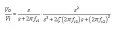# Third order high pass filter design

#### connellyw

Joined Aug 2, 2016
6
Hi All,
Just need a little push in the right direction.
We have to design a third order high pass filter, which I've calculated all resistors and caps for. We had to use 2 x LM108 IC's, which I understand makes the circuit less than ideal.

Using my theoretical pole positions, I need to plot magnitude and phase and group delay. This should be a bode plot, correct?

So I need to calculate a transfer function (which should be a stage 1 TF multiplied by a stage 2 TF) to plot this in matlab. correct?

I've calculated first stage cutoff freq, second stage cutoff freq and ς, so I should be able to use the attached formula, correct?Or is there an easier way to do this?

Any pointers would be great, thanks!

#### Papabravo

Joined Feb 24, 2006
20,378
Was there a design criteria such as maximal flatness or maximum ripple or something else that would give us a clue as to what you are doing. Your question implies that there is a unique 3rd order filter design algorithm and that is manifestly NOT the case.

#### connellyw

Joined Aug 2, 2016
6
Was there a design criteria such as maximal flatness or maximum ripple or something else that would give us a clue as to what you are doing. Your question implies that there is a unique 3rd order filter design algorithm and that is manifestly NOT the case.
Hi,
No, but the only thing ive worked out is that a bessel filter has no measureable ripple.

Whats the generic third order high pass algorithm?

#### MrAl

Joined Jun 17, 2014
10,592
Hi,
No, but the only thing ive worked out is that a bessel filter has no measureable ripple.

Whats the generic third order high pass algorithm?

Hello,

The first order is:
(s*R*C)/(s*R*C+1)

or rewritten:
s/(s+1/RC)

or rewritten again as:
s/(s+T)

where T is 1/(R*C).

If one stage is s/(s+T), then three isolated stages in cascade would be:
[s/(s+T)]^3

and that would be as:
Vin o---stage1---stage2---stage3---oVout

so we get s^3/(s+T)^3.

That's your basic third order high pass filter.

If the filter is all passive (no op amps) then the stages interact with each other so we get a somewhat different expression, but if each stages has a progressively higher impedance then it does not load the preceding stage that much so we still get that approximate response.

With all different components we have for the passive filter:
Vout=(s^3*Vin*C1*C2*C3*R1*R2*R3)/(s^3*C1*C2*C3*R1*R2*R3+s^2*C2*C3*R2*R3+s^2*
C2*C3*R1*R3+s^2*C1*C3*R1*R3+s*C3*R3+s^2*C2*C3*R1*R2+s^2*C1*C3*R1*R2+s^2*C1*C2*R1*R2+
s*C3*R2+s*C2*R2+s*C2*R1+s*C1*R1+1)

With 10x and 100x impedance scaling for the second and third stages we have:
Vout=(1000000*s^3*Vin*C1^3*R1^3)/(1000000*s^3*C1^3*R1^3+1121100*s^2*C1^2*R1^2+11111*s*C1*R1+1)

and finally with all C and R the same value we have:
Vout=(s^3*Vin*C1^3*R1^3)/(s^3*C1^3*R1^3+6*s^2*C1^2*R1^2+5*s*C1*R1+1)

and if we let T=R*C this time we get:
Vout=(s^3*Vin*T^3)/(s^3*T^3+6*s^2*T^2+5*s*T+1)

and if we let T=1/(R*C) this time we get:
Vout=(s^3*Vin)/(T^3+5*s*T^2+6*s^2*T+s^3)

Last edited:

#### Papabravo

Joined Feb 24, 2006
20,378
Hi,
No, but the only thing ive worked out is that a bessel filter has no measureable ripple.

Whats the generic third order high pass algorithm?
That is not true. It is the Butterworth filter that has maximal flatness in gain or attenuation. The Bessel filter maximizes the flatness of the group delay of the filter at zero frequency. AFAIK, there is no generic 3rd order, or any other order, filter. Each filter is designed to specifications about required attenuation in the passband, transition band, and stopband. Additionally you can specify the allowed ripple and other characteristics. Most filters are a compromise and attempt to meet one or more objective specifications. That is why I asked the question the way I did.

#### MrAl

Joined Jun 17, 2014
10,592
Hello again,

I forgot to mention that the capacitor ESR is more important in evaluating the high pass filter because it creates a low pass filter effect even though we dont really want that. This must be considered when evaluating the filters. The ESR is not shown in the formulations i provided, but we can add them if you feel you want to evaluate the filters in a more realistic way. The idea is to add a small value resistance in series with each capacitor and then redo the formulation.